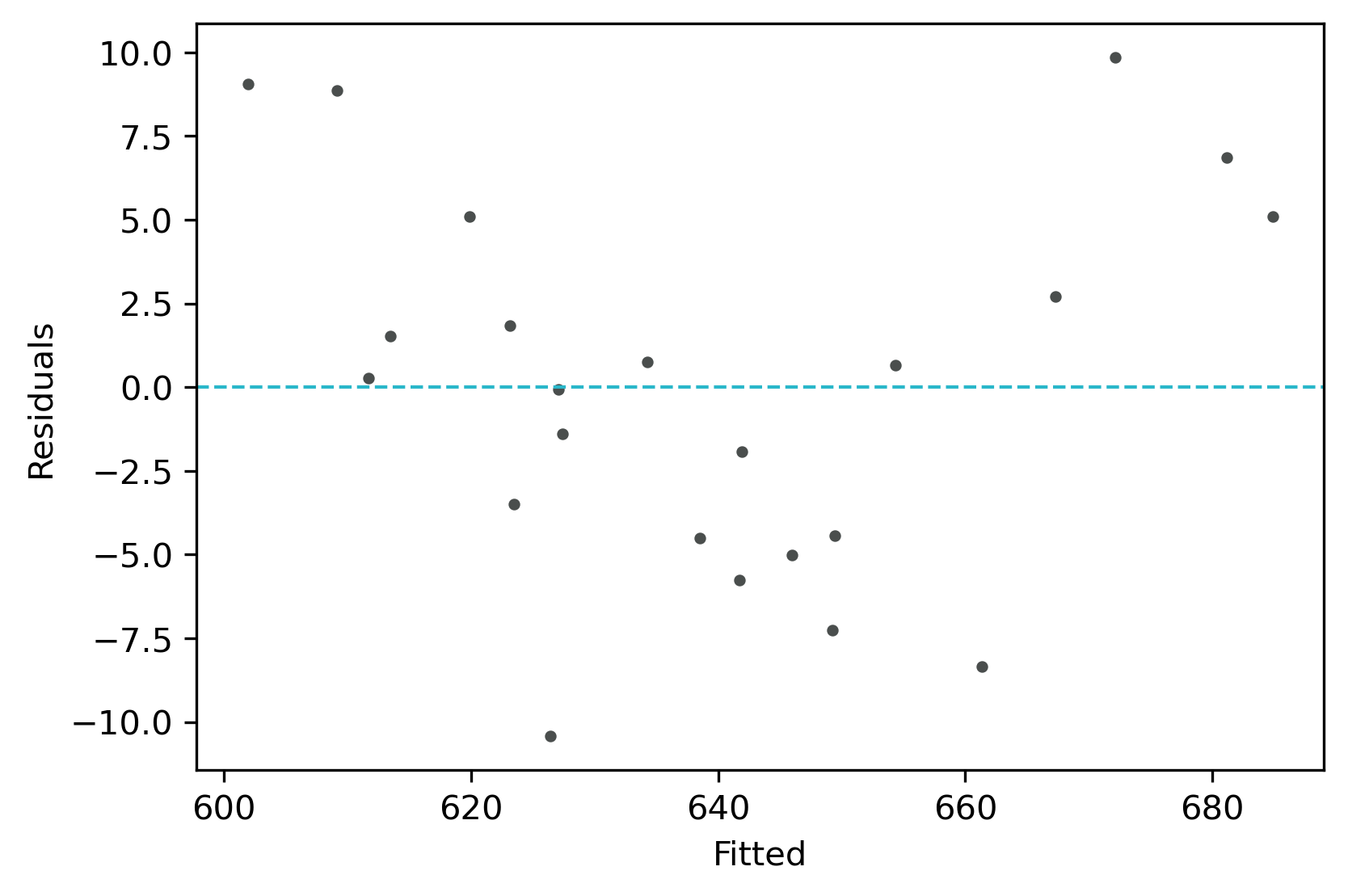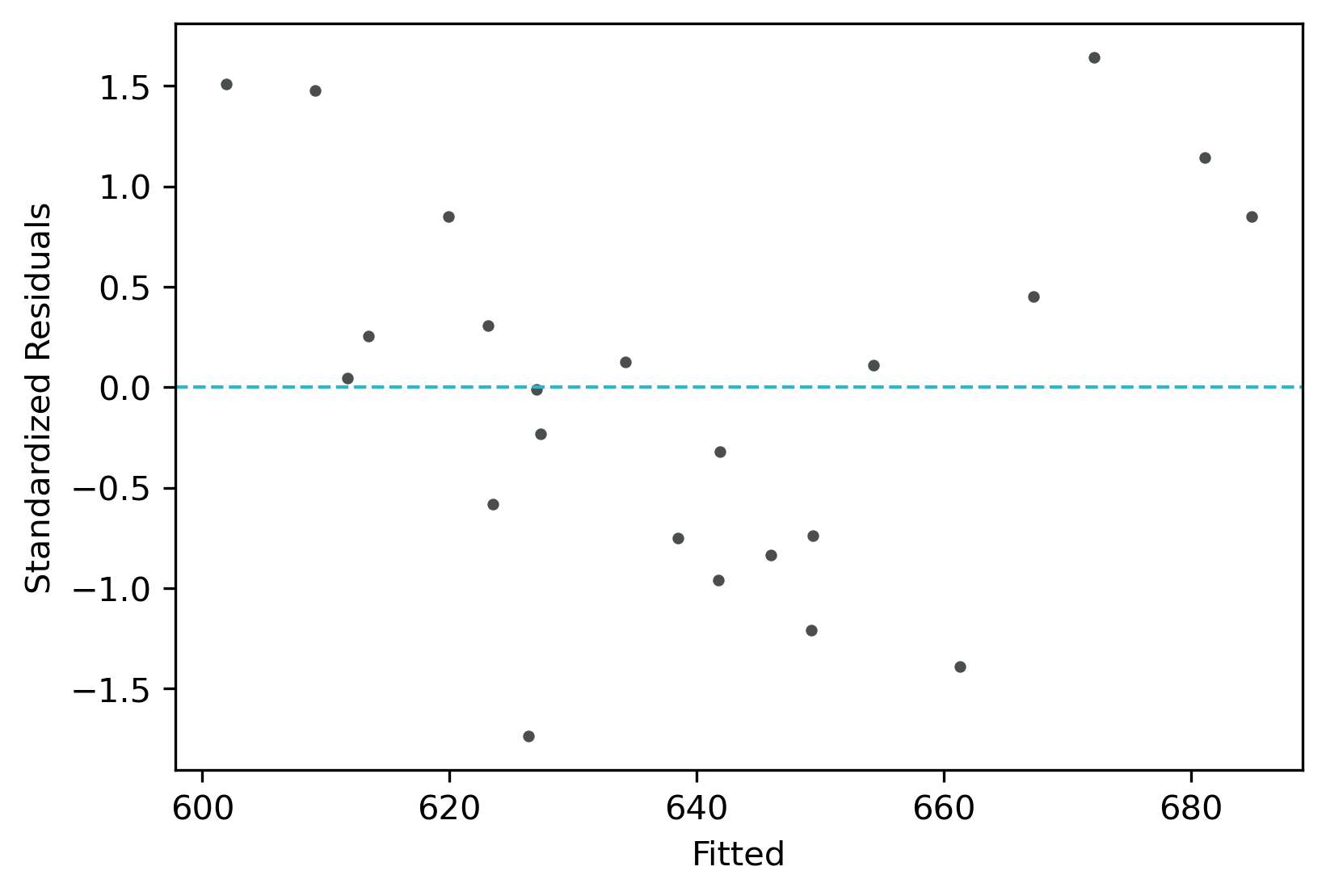# Multiple linear regression (MLR)

## Multiple Linear Regression (MLR)?

• In MLR, linear relationships between Y (dependent/response/criterion/endogeneous) and X (explanatory/independent/predictor/regressor/exogeneous) variables can be explained by multiple X variables

## Multiple Linear Regression in Python

``````# you can use interactive python interpreter, jupyter notebook, google colab, spyder or python code
# I am using interactive python interpreter (Python 3.8.2)
>>> from bioinfokit.analys import stat, get_data
# load dataset as pandas dataframe. it should not have missing values.
>>> df = get_data('mlr').data
X1   X2    Y
0  25  355  670
1  30  398  690
2  18  328  635
3  15  320  625
4  20  335  640

>>> reg = stat()
>>> reg.lin_reg(df=df, x=['X1', 'X2'], y=['Y'])

Regression equation:

521.4505 + (3.258*X1) + (0.1651*X2)

Regression Summary:

----------------------------------------  ------------
Dependent variables                       ['X1', 'X2']
Independent variables                     ['Y']
Coefficient of determination (r-squared)  0.9425
Root Mean Square Error (RMSE)             5.5869
Mean of Y                                 639.3913
Residual standard error                   5.989
No. of Observations                       23

Regression Coefficients:

Parameter      Estimate    Std Error    t-value    P-value Pr(>|t|)
-----------  ----------  -----------  ---------  ------------------
Intercept    521.45          16.825    30.9926           2.2167e-18
X1             3.25799        0.2694   12.0935           1.1852e-10
X2             0.165104       0.0565    2.92219          0.0084258

ANOVA Summary:

Source      Df    Sum Squares  Mean Squares    F         Pr(>F)
--------  ----  -------------  --------------  --------  ----------
Model        2      11768.1    5884.0544       164.0453  3.9208E-13
Error       20        717.369  35.8685
Total       22      12485.5

Variance inflation factor (VIF)

Variable        VIF
----------  -------
X1          1.64824
X2          1.64824

``````

### Interpretation

• The regression line with equation [Y = 521.4505 + (3.258X1) + (0.1651X2)], is helpful to predict the value of the Y variable from the given value of the X variables (X1 and X2). In general, a regression can be useful in predicting the Y of any value within the range of X. It can be also used to predict Y from X outside the given range, but such extrapolation may not be useful
• The slope (3.25799 and 0.165104) represents the change in the Y per unit change in the X variable when other X variables remain constant. It means that the value Y increases by 3.25799 with each unit increase in X1 when X2 is constant. Similarly, value Y increases by 0.165104 with each unit increase in X2 when X1 is constant. The regression coefficients should be interpreted confidently only if they are statistically significant (P<0.05)
• The intercept (521.45) represents the value of Y when all X variables have a value of 0 (X1=0 and X2=0). Here need to be cautious to interpret intercept as sometimes the value (X1=0) does not make any sense (e.g. speed of car or height of the person). In such cases, the values within the range of X should be considered to interpret the intercept
• The coefficient of determination (r-squared) is 0.918 (91.8%), which suggests that 91.8% of the variance in Y can be explained by X variables (X1 and X2). In MLR, adjusted r-squared is more appropriate than r-squared as an increasing number of X variables also increases r-squared
• From Regression Coefficients, the P-value obtained for X1 and X2 is significant (<0.05), it suggests that X1 and X2 significantly influence the response variable Y (there is a significant relationship between X and Y)
• From ANOVA, the P-value is significant (<0.05), which suggests that there is a significant relationship between X and Y. The X variables can reliably predict the Y variable
• In MLR, multicollinearity (the relationship between the X variables) is often a problem. It causes inflation of variance, changes in the signs and confidence intervals of regression coefficients (Ryan, 2008). Therefore, it is necessary to detect and eliminate multicollinearity. Multicollinearity can be detected using Variance inflation factor (VIF)
• Generally, VIF > 5 or > 10 suggests the multicollinearity (Vatcheva et al., 2016). But this is not a universal agreement and VIF < 5 could also suggest the multicollinearity (Vatcheva et al., 2016)
• If VIF < 2 is a good indicator of the absence of strong multicollinearity and VIF = 1 indicates completely absence of multicollinearity
• Sometimes, a correlation coefficient > 0.5 among the X variables can be used to detect the multicollinearity (Vatcheva et al., 2016)
• To remove the multicollinearity you can either take more measurements or remove the variables causing multicollinearity or perform the ridge regression (Ryan, 2008)
• In MLR result, the VIF for both of the X variables (X1=1.64824 and X2=1.64824) are < 2 and suggests there is no strong relationship between the X variables

### Check Multiple Linear Regression (MLR) Assumptions

Residuals vs fitted (y_hat) plot: This plot used to check for linearity, variances and outliers in the regression data

``````# get residuals and standardized residuals and add to original dataframe
>>> df['yhat']=reg.y_hat
>>> df['res']=reg.residuals
>>> df['std_res']=reg.std_residuals
X1   X2    Y        yhat       res   std_res
0  25  390  670  667.290604  2.709396  0.451566
1  30  398  690  684.901388  5.098612  0.849769
2  18  328  635  634.248248  0.751752  0.125292
3  15  320  625  623.153447  1.846553  0.307759
4  20  335  640  641.919955 -1.919955 -0.319993

# create fitted (y_hat) vs residuals plot
>>> visuz.stat.reg_resid_plot(df=df, yhat='yhat', resid='res', stdresid='std_res')
# plot will be saved in same dir (resid_plot.png and std_resid_plot.png)
# set parameter show=True, if you want view the image instead of saving
``````From the plot,

• As the data is pretty equally distributed around the line=0 in the residual plot, it meets the assumption of residual equal variances. The outliers could be detected here if the data lies far away from the line=0.
• In the standardized residual plot, the residuals are within -2 and +2 range and suggest that it meets assumptions of linearity

Quantile-quantile (QQ) plot: This plot used to check the data normality assumption

``````>>> import statsmodels.api as sm
>>> import matplotlib.pyplot as plt
# check dataframe for required variables (we will need std_res variable for QQ plot)
X1   X2    Y        yhat       res   std_res
0  25  390  670  667.290604  2.709396  0.451566
1  30  398  690  684.901388  5.098612  0.849769
2  18  328  635  634.248248  0.751752  0.125292
3  15  320  625  623.153447  1.846553  0.307759
4  20  335  640  641.919955 -1.919955 -0.319993

# create QQ plot
# line=45 option to plot the data around 45 degree line
>>> sm.qqplot(df['std_res'], line='45')
>>> plt.xlabel("Theoretical Quantiles")
>>> plt.ylabel("Standardized Residuals")
>>> plt.show()

``````From the plot,

• As the standardized residuals lie around the 45-degree line, it suggests that the residuals are normally distributed

## Multiple linear regression with PyTorch

Let’s perform a MLR with PyTorch with the same dataset

``````>>> from bioinfokit.analys import stat, get_data
>>> import torch as th
>>> df = get_data('mlr').data
X1   X2    Y
0  25  390  670
1  30  398  690
2  18  328  635
3  15  320  625
4  20  335  640

# convert to PyTorch tensor
# variable shape should be (samples, features)
>>> X = th.tensor(df[['X1', 'X2']].values, dtype=th.float32)
>>> Y = th.tensor(df[['Y']].values, dtype=th.float32)

# MLR model using PyTorch
>>> in_features = 2 # number of independent variable
>>> out_features = 1 # dim of predicted variable
>>> mlr_model = th.nn.Linear(in_features, out_features)

# define loss function (regression error)
>>> mse_loss = th.nn.MSELoss()
# optimize to minimize the loss function and find optimal LR parameters (Regression Coefficients)
# set number of iterations until you see the convergence in the loss function
# when you see similar minimum values for a large number of ending iterations
# it will give you the best values of LR parameters
>>> n_iter = 25000
>>> for i in range(n_iter):
# predict model with current MLR parameters
y_pred = mlr_model(X)
# calculate loss function
step_loss = mse_loss(y_pred, Y)

# Backward to find the derivatives of the loss function with respect to LR parameters
# make any stored gradients to zero
step_loss.backward()
# update with current step LR parameters
optimizer.step()

print ('i [{}], Loss: {:.2f}, Bias {:.2f}'.format(i, step_loss.item(), mlr_model.bias.item()))

# see the last few iterations
i , Loss: 31.36, Bias 521.42
i , Loss: 31.26, Bias 521.42
i , Loss: 31.19, Bias 521.42
i , Loss: 31.22, Bias 521.42
i , Loss: 31.28, Bias 521.42
i , Loss: 31.30, Bias 521.42
i , Loss: 31.26, Bias 521.42
i , Loss: 31.20, Bias 521.42
i , Loss: 31.19, Bias 521.42
i , Loss: 31.23, Bias 521.42
i , Loss: 31.25, Bias 521.42
i , Loss: 31.24, Bias 521.42
i , Loss: 31.21, Bias 521.42
i , Loss: 31.19, Bias 521.42

# bias parameter converged  here to 521.42 for last few iterations
# also loss is also converged
``````

``````# intercept (a)
>>> mlr_model.bias.item()
521.4164428710938
# slope (b)
>>> mlr_model.weight.detach()
tensor([[3.2580, 0.1655]])
``````

Now, let’s predict the Y from some random X1 and X2,

``````>>> X1_data, X2_data = 28, 396
# predict Y value when X1 is 28 and X2 is 396
>>> y_pred = mlr_model(th.tensor([[X1_data, X2_data]], dtype=th.float32)).detach()
>>> y_pred.item()
678.1707763671875
# Y=678.17 when X1 is 28 and X2 is 396
``````
• Vatcheva KP, Lee M, McCormick JB, Rahbar MH. Multicollinearity in regression analyses conducted in epidemiologic studies.
Epidemiology (Sunnyvale, Calif.). 2016 Apr;6(2).
• Ryan TP. Modern regression methods. John Wiley & Sons; 2008 Nov 10.

How to cite?
Renesh Bedre.(2020, July 29). reneshbedre/bioinfokit: Bioinformatics data analysis and visualization toolkit (Version v0.9). Zenodo. http://doi.org/10.5281/zenodo.3965241

If you have any questions, comments or recommendations, please email me at reneshbe@gmail.com

Last updated: July 18, 2020